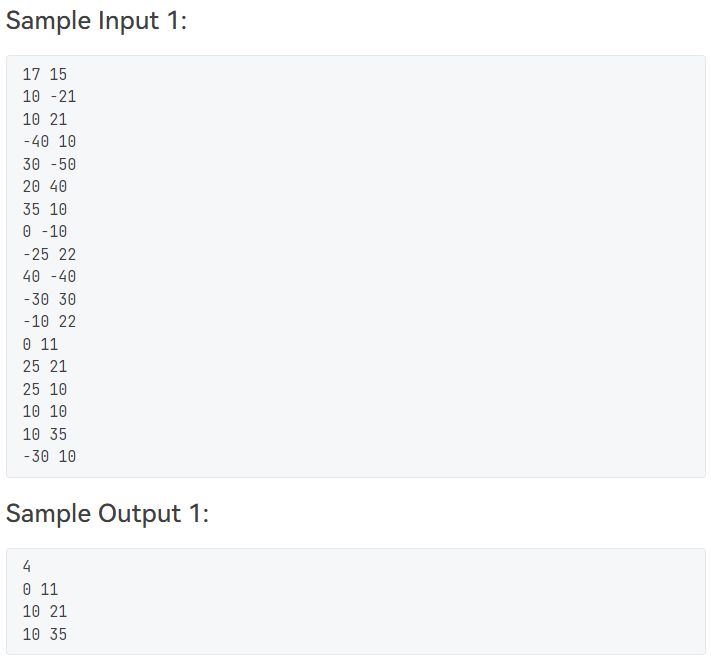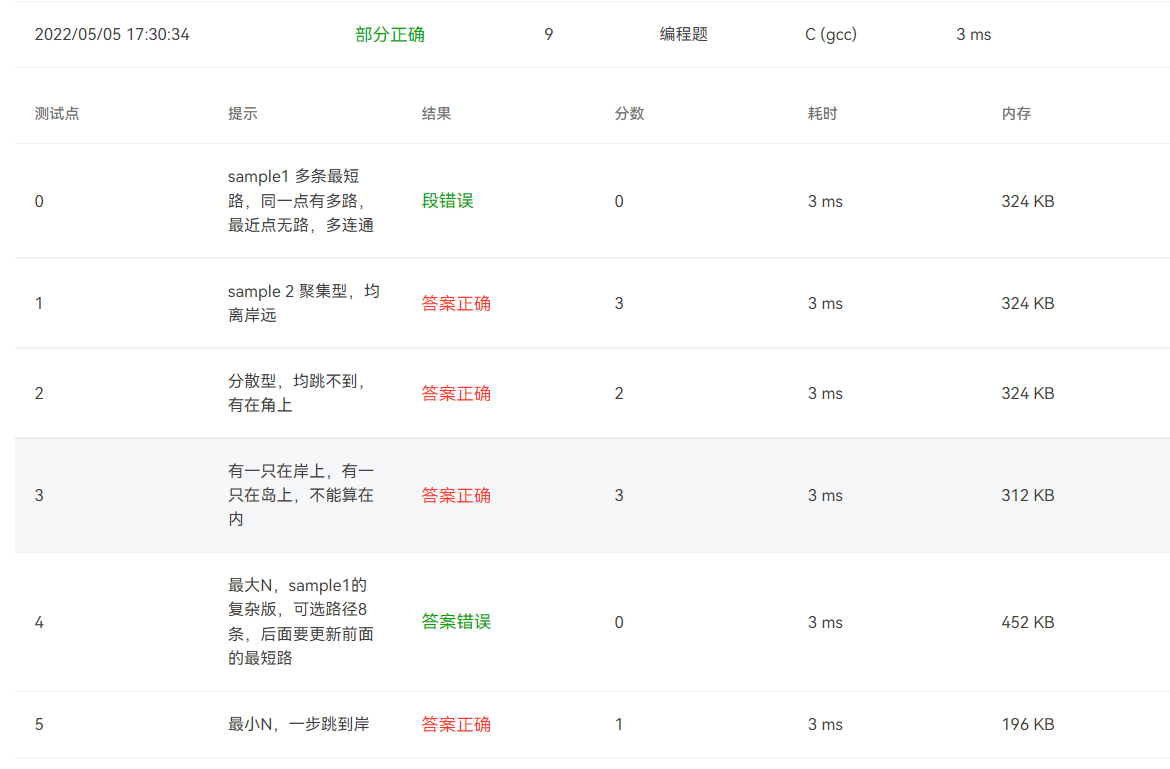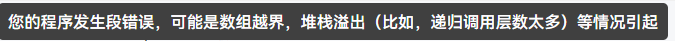浏览 46

# 数据结构习题，图的Dijkstra算法

###### 题目如下

This time let us consider the situation in the movie "Live and Let Die" in which James Bond, the world's most famous spy, was captured by a group of drug dealers. He was sent to a small piece of land at the center of a lake filled with crocodiles. There he performed the most daring action to escape -- he jumped onto the head of the nearest crocodile! Before the animal realized what was happening, James jumped again onto the next big head（省略号） Finally he reached the bank before the last crocodile could bite him (actually the stunt man was caught by the big mouth and barely escaped with his extra thick boot).

Assume that the lake is a 100 by 100 square one. Assume that the center of the lake is at (0,0) and the northeast corner at (50,50). The central island is a disk centered at (0,0) with the diameter of 15. A number of crocodiles are in the lake at various positions. Given the coordinates of each crocodile and the distance that James could jump, you must tell him a shortest path to reach one of the banks. The length of a path is the number of jumps that James has to make.

Input Specification:
Each input file contains one test case. Each case starts with a line containing two positive integers N (≤100), the number of crocodiles, and D, the maximum distance that James could jump. Then N lines follow, each containing the (x,y) location of a crocodile. Note that no two crocodiles are staying at the same position.

Output Specification:
For each test case, if James can escape, output in one line the minimum number of jumps he must make. Then starting from the next line, output the position (x,y) of each crocodile on the path, each pair in one line, from the island to the bank. If it is impossible for James to escape that way, simply give him 0 as the number of jumps. If there are many shortest paths, just output the one with the minimum first jump, which is guaranteed to be unique.###### 我的代码
``````//自己写的，半错版本
#include<stdio.h>
#include<stdlib.h>
#include<math.h>

#define MaxVertexNum 105
#define M_INFINITY 65535
typedef int Vertex;
typedef double WeightType;
typedef struct Data{
int X,Y;
}DataType[MaxVertexNum];

/*边的定义*/
typedef struct ENode* PtrToENode;
struct ENode{
Vertex V1,V2;
WeightType Weight;
};
typedef PtrToENode Edge;

/*图的定义*/
typedef struct GNode* PtrToGNode;
struct GNode{
int Nv;
int Ne;
WeightType D[MaxVertexNum][MaxVertexNum];
DataType Locate;
};
typedef PtrToGNode MGraph;

//创造图的函数
void InsertEdge(MGraph Graph, Edge E)
{
Graph->D[E->V1][E->V2] = E->Weight;
Graph->D[E->V2][E->V1] = E->Weight;
}
MGraph CreatGraph(int VertexNum)
{/*初始化一个图结构，所有对角边为0，非对角边为INFINITY*/
MGraph Graph = (MGraph)malloc(sizeof(struct GNode));
Graph->Nv = VertexNum;
Graph->Ne = 0;
int V,W;
for(V=0;V<Graph->Nv;V++){
for(W=V;W<Graph->Nv;W++){
if(V!=W){
Graph->D[V][W] = M_INFINITY;
Graph->D[W][V] = M_INFINITY;
}else{
Graph->D[V][W] = 0;
}
}
}
return Graph;
}
WeightType Length(double X1,double Y1,double X2,double Y2)
{/*返回两个点的直线距离*/
double deltx = X1-X2;
double delty = Y1-Y2;
WeightType weight = sqrt(deltx*deltx + delty*delty);
return weight;
}
MGraph BuildGraph(int Nv,double D)
{/*创造图*/
int i,V,W;
Vertex X,Y;
WeightType Weight;
MGraph Graph = CreatGraph(Nv+1);
Edge E = (Edge)malloc(sizeof(struct ENode));

V=1;
Graph->Locate.X = Graph->Locate.Y = 0;
for(i=1;i<=Nv;i++){
scanf("%d%d",&X,&Y);

if(abs(X)<50 && abs(Y)<50 && Length(X,Y,0,0)>7.5){
Graph->Locate[V].X = X;
Graph->Locate[V].Y = Y;
V++;
}else{
Graph->Nv--;
}
}

for(V=0;V<Graph->Nv;V++){
for(W=V+1;W<Graph->Nv;W++){
Weight = Length(Graph->Locate[V].X,Graph->Locate[V].Y,
Graph->Locate[W].X,Graph->Locate[W].Y);
if(Weight<=D){
E->V1 = V;
E->V2 = W;
E->Weight = Weight;
if(E->V1==0) E->Weight-=7.5;
InsertEdge(Graph,E);
}
}
}

return Graph;
}

Vertex FindMinDist(MGraph Graph, double dist[], int collected[])
{
Vertex MinV, V;
int MinDist = M_INFINITY;

for(V=0; V<Graph->Nv; V++){
if(collected[V]==0 && dist[V]<MinDist){
MinDist = dist[V];
MinV = V;
}
}
if( MinDist < M_INFINITY )
return MinV;
else return 0;
}
void Dijkstra(MGraph Graph, double* dist, int* path,int* collected)
{
Vertex V,W;

for(V=0;V<Graph->Nv;V++){
dist[V] = Graph->D[V];
if(dist[V]<M_INFINITY)
path[V] = 0;
else
path[V] = -1;
collected[V] = 0;
}
/*先将起点收入集合*/
dist = 0;
collected = 1;

while(1){
V = FindMinDist(Graph,dist,collected);
if(V==0)
break;
collected[V] = 1;
for(W=0;W<Graph->Nv;W++)
if(collected[W]==0 && Graph->D[V][W]<M_INFINITY){
if(dist[V]+Graph->D[V][W]<dist[W]){
dist[W] = dist[V]+Graph->D[V][W];
path[W] = V;
}
}
}
}

int min(int x,int y)
{
if(x<y) return x;
else return y;
}

int main()
{
/*输入数，（初始Nv，可能会更少），输入JanesBond一次最远跳*/
int N,D,i;
scanf("%d%d",&N,&D);
/*创造图*/
MGraph Graph = BuildGraph(N,D);
/*初始化dist[],path[],collected[]*/
double dist[Graph->Nv];
int path[Graph->Nv],collected[Graph->Nv];
for(i=0;i<Graph->Nv;i++){
dist[i] = M_INFINITY;
path[i] = -1;
collected[i] = 0;
}
/*Dijkstra算法算dist*/
Dijkstra(Graph,dist,path,collected);

/*找到可以作为出口得鳄鱼，同时将它到出口的距离存储到数组中*/
Vertex V,W,OutNode[Graph->Nv];
int flag = 0;
for(V=0;V<Graph->Nv;V++) OutNode[V]=0;
for(V=0;V<Graph->Nv;V++){
if(50-fabs(Graph->Locate[V].X)<=D || 50-fabs(Graph->Locate[V].Y)<=D){
if(50-fabs(Graph->Locate[V].X)<=D){
OutNode[V] = 50-fabs(Graph->Locate[V].X);
}else if(50-fabs(Graph->Locate[V].Y<=D)){
OutNode[V] = 50-fabs(Graph->Locate[V].X);
}
flag=1;
}
}
if(!flag){
printf("0");
return 0;
}
flag = 0;
for(W=1;W<Graph->Nv;W++){
if(Graph->D[W]!=M_INFINITY){
flag = 1;
}
}
if(!flag){
printf("0");
return 0;
}
/*以上为判断是否有可能可以出去，也就是1>Island有没有邻接点，2>有没有可以跳出去的鳄鱼*/

/*
找最短路
1.找可以出去的路径
2.比较可以出去的路径中最短路径
*/

Vertex OutWay[Graph->Nv];
for(i=0;i<Graph->Nv;i++){
OutWay[i]=-1;
}
for(i=0;i<Graph->Nv;i++){
if(50-abs(Graph->Locate[i].X)<=D || 50-abs(Graph->Locate[i].Y)<=D){
OutWay[i] = min(50-abs(Graph->Locate[i].X),50-abs(Graph->Locate[i].Y));
}
}

/*从出口中更新路径长度*/
double Outdist[Graph->Nv];
for(i=0;i<Graph->Nv;i++){
Outdist[i] = 0;
}
for(i=0;i<Graph->Nv;i++){
if(OutWay[i]!=-1){
Vertex temNode = i;
while(temNode){
Outdist[i]+=dist[temNode];
temNode = path[temNode];
}
}
}

/*从路径中找最小长，并记录*/
Vertex MinWay;
double Mindist=M_INFINITY;
for(i=0;i<Graph->Nv;i++){
if(OutWay[i]!=-1){
if(Mindist>Outdist[i]){
Mindist = Outdist[i];
MinWay = i;
}
}
}

/*从最小路径中找结点，反向输出*/
Vertex Stack[Graph->Nv];
int rear=-1,count=1;
Vertex temNode = MinWay;
while(temNode){
Stack[++rear] = temNode;
temNode = path[temNode];
count++;
}
printf("%d\n",count);
while(rear!=-1){
printf("%d %d\n",Graph->Locate[Stack[rear]].X,Graph->Locate[Stack[rear]].Y);
rear--;
}

return 0;
}

``````
###### 最后结果• 写回答

#### 2条回答默认 最新

•关注

段错误一般是数组下标越界
或者指针没有正确指向或没有分配空间
参考代码

``````#include<bits/stdc++.h>
using namespace std;
struct Node {
double x, y;
double distant;
int c;
};
struct TS {
int num;
double distant;
};

TS HHH;
int N, path;
double D, G;
Node Dist;
bool visit = {false};

void Init(int i, int j);
int BFS(int i);
int Judge(int i);
bool cmp(TS a, TS b) {
return a.distant < b.distant;
}

int main() {
cin >> N >> D;
if (D >= 32.5) {
printf("1\n");
return 0;
}
int X, Y;
int tol = 0;
fill(G, G + 105*105, 200);
for (int i = 0; i < N; i++) {
cin >> X >> Y;
HHH[i].num = i;
Dist[i].x = X;
Dist[i].y = Y;
Dist[i].distant = sqrt(X * X + Y * Y);
HHH[i].distant = Dist[i].distant;
Dist[i].c = 0;
path[i] = -1;
}
for (int i = 0; i < N - 1; i++) {
for (int j = i + 1; j < N; j++) {
Init(i, j);
}
}

sort(HHH, HHH + N, cmp);

for (int i = 0; i < N; i++) {
if (HHH[i].distant > 7.5 && HHH[i].distant <= D + 7.5 && !visit[HHH[i].num]) {
tol = BFS(HHH[i].num);
if (tol > 1) break;
}
}
if (tol == 0) printf("0\n");
return 0;
}

void Init(int i, int j) {
int x = Dist[i].x - Dist[j].x;
int y = Dist[i].y - Dist[j].y;
G[i][j] = G[j][i] = sqrt(x * x + y * y);
}

int Judge(int i) {
if ((Dist[i].x + D >= 50) || (Dist[i].x - D <= -50) || (Dist[i].y + D >= 50) || (Dist[i].y - D <= -50)) return 1;
else return 0;
}

int BFS(int i) {

visit[i] = true;
Dist[i].c = 1;
queue<int> Q;
Q.push(i);

while (!Q.empty()) {
int temp = Q.front();
Q.pop();
if (Judge(temp)) {
cout << Dist[temp].c + 1 << endl;
stack<int> st;
while (temp != -1) {
st.push(temp);
temp = path[temp];
}
while (!st.empty()) {
int Top = st.top();
st.pop();
cout << Dist[Top].x << " " << Dist[Top].y << endl;
}
return 2;
}
for (int i = 0; i < N; i++) {
if (G[temp][i] <= D && !visit[i]) {
visit[i] = true;
Dist[i].c = Dist[temp].c + 1;
path[i] = temp;
Q.push(i);
}
}
}
return 0;
}

``````
本回答被题主选为最佳回答 , 对您是否有帮助呢?
评论 编辑记录

#### 悬赏问题

• ¥15 arm虚拟机无法和物理机互通
• ¥15 如何在此代码上增加一个统计学生生源的功能？(语言-c语言)
• ¥15 Android导航条遮盖异常
• ¥15 计算机网络技术基础问题
• ¥15 设置mac系统只能访问指定网站
• ¥15 西门子博途 s7 1200控制三台步进电机
• ¥15 基于非参数的方向距离函数求污染物影子价格(有偿)
• ¥15 vue+element 生成table
• ¥15 实验 4 FIFO 算法和 LRU 算法-C 程序实现
• ¥15 有偿拼接大疆精灵4RGB影像